# Let's suppose that a firm's production technology is represented by the following production function q =...

Let's suppose that a firm's production technology is represented by the following production function

q = F( K, L) = min { 2K, L }

What is the MRTS when 2K > L

a. Infinite

b. 0

c.

1/2

d. 2

What is the MRTS whena. 0

b. 1/3

c. infinite

d. 2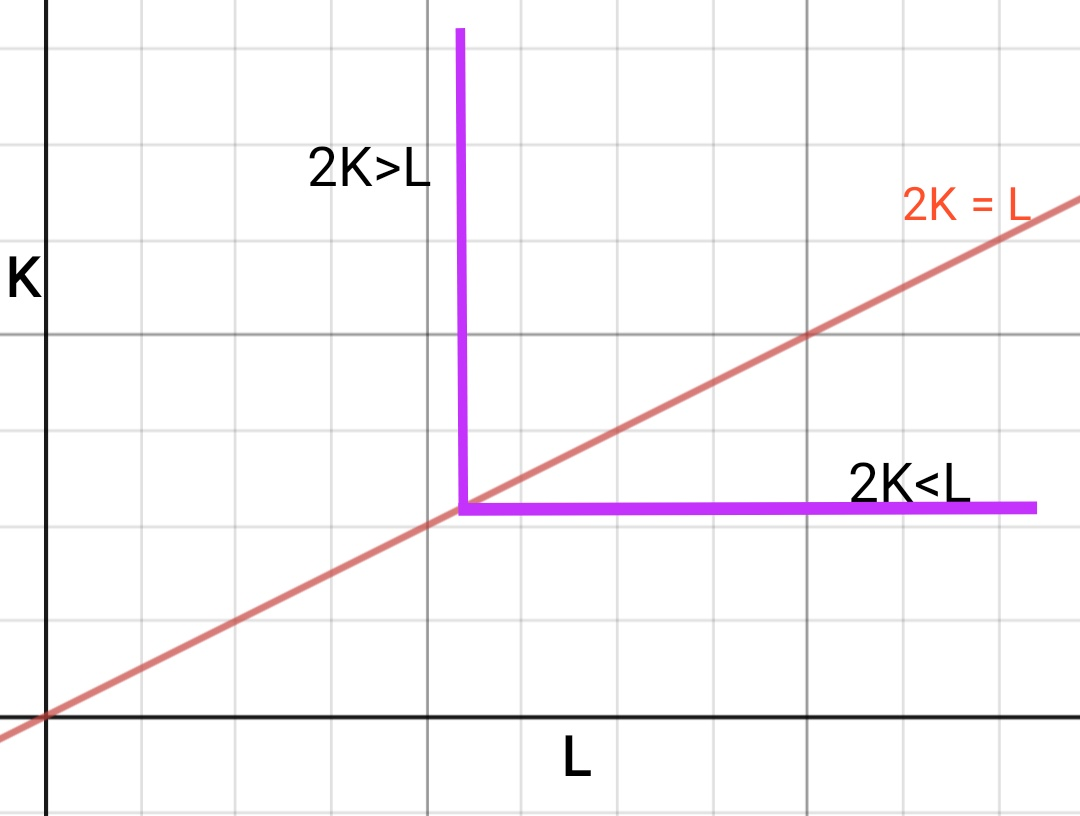Pink color L shaped curve is IsoQuant

So MRTS is Slope of isoquant

A) option A) infinite

when 2K> L, then Vertical segment of IC

So Slope of Isoquant = infinity

.

B) option A) zero

if 2K < L

Then horizontal segment,

So Slope = 0

#### Earn Coin

Coins can be redeemed for fabulous gifts.

Similar Homework Help Questions
• ### (1) Let's suppose that a firm's production technology is represented by the following production function. What...

(1) Let's suppose that a firm's production technology is represented by the following production function. What is the marginal product of labor (MPL)? a. -3 b. 1 c. 3 d. 1/3 What is the marginal product of capital (MPK)? a. -1 b. 1/3 c. 3 d. 1 What is the marginal rate of technical substitution (MRTS)? a. 1/3 b.3 c. -1/3 d. 1

• ### Let's suppose that the current state of a firm's production technology is represented by the following...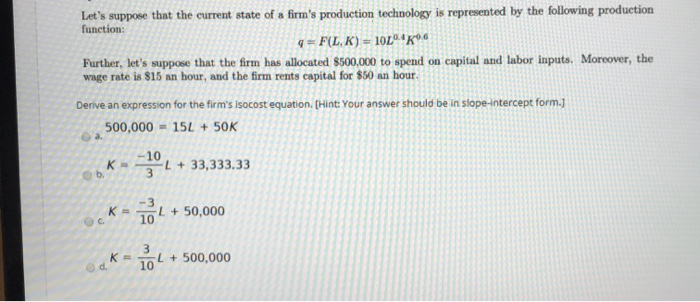Let's suppose that the current state of a firm's production technology is represented by the following production function: 4F(LK) = 1024K06 Further, let's suppose that the firm has allocated \$500,000 to spend on capital and labor inputs. Moreover, the wage rate is \$15 an hour, and the firm rents capital for \$50 an hour. Derive an expression for the firm's Isocost equation. (Hint: Your answer should be in slope-intercept form.] 500,000 = 15L + 50K 10L + 33,333.33 L +...

• ### 7. Suppose a firm's production function is Q-min(L,K). (This means the level of O produced is...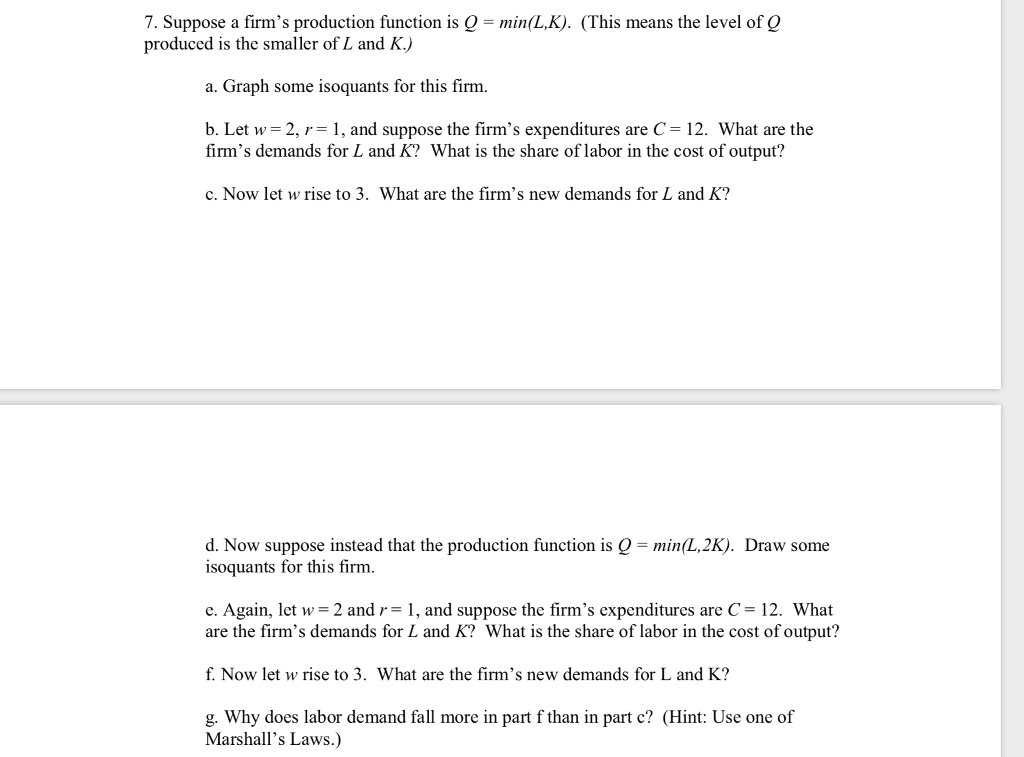7. Suppose a firm's production function is Q-min(L,K). (This means the level of O produced is the smaller of L and K) a. Graph some isoquants for this firm. b. Let w = 2, r= 1, and suppose the firm's expenditures are C-12. What are the firm's demands for L and K? What is the share of labor in the cost of output? c. Now let w rise to 3. What are the firm's new demands for L and K?...

• ### 5. Suppose that your production function is q = K + L. If the firm is able to buy all the capital and labor it wants at...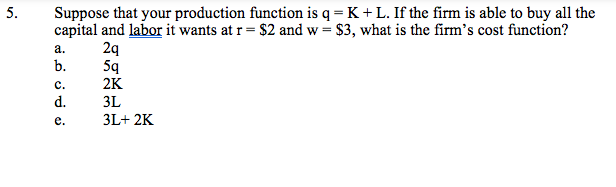5. Suppose that your production function is q = K + L. If the firm is able to buy all the capital and labor it wants at r = \$2 and w \$3, what is the firm's cost function? 2q 5q 2K a. b. C. d. 3L 3L+ 2K e. 5. Suppose that your production function is q = K + L. If the firm is able to buy all the capital and labor it wants at r = \$2...

• ### Course Materials - 202001-12752 https://bb. 1. A firm has the following production function: 4 = 2L"2114...Course Materials - 202001-12752 https://bb. 1. A firm has the following production function: 4 = 2L"2114 a. Suppose K= l in the short run but L is variable. Let w denote the wage rate and letr denote the rental rate of capital. Solve for the firm's short run production function and short run cost function. (3 points). b. What is the marginal cost of an additional unit of g in the short run? Is it increasing, decreasing, or constant in...

• ### 1. Suppose that a firm's production function of output Q is a function of only two...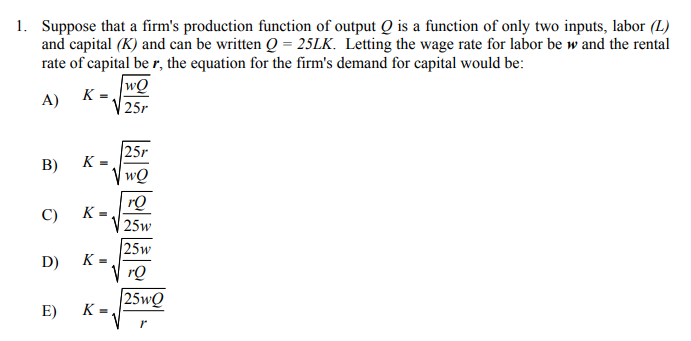1. Suppose that a firm's production function of output Q is a function of only two inputs, labor (L) and capital (K) and can be written Q = 25LK. Letting the wage rate for labor be w and the rental rate of capital be r, the equation for the firm's demand for capital would be: wQ A) K = 25r B) K = C) K- 25r wQ rQ 25w 25w rQ 25wQ D) E) KE

• ### For each of the following production functions calculate the ( MRTS v L,K ) a. Q...

For each of the following production functions calculate the ( MRTS v L,K ) a. Q = L^2/3 K^1/3 when Q=8 b. Q = 3L + K when Q=3 c. Q = min{3L, K} when Q=3

• ### Suppose the firm's production function is Q = 2KL where Q is units of output, K...

Suppose the firm's production function is Q = 2KL where Q is units of output, K is units of capital (which are fixed at 2), and L is units of labor. a. What is the firm’s short-run production function? b. Over the labor input usage range of 0 to 5, that is L ranging from 0 to 5, graph the firm’s Total Product curve. c. Derive and graph the firm’s Average Product curve and the Marginal Product curve. Graph/plot them...

• ### Suppose the production function of a firm is given by q = L1/4K1/4. The prices of...

Suppose the production function of a firm is given by q = L1/4K1/4. The prices of labor and capital are given by w = \$10 and r = \$20, respectively. a) Write down the firm's cost minimization problem. b) What returns to scale does the production function exhibit? Explain c) What is the Marginal Rate of Technical Substitution (MRTS) between capital and labor? d) What is the optimal capital to labor ratio? Show your work. e) Derive the long run...

• ### 8. Suppose that the following graph shows a few of the isoquants for a firm's production...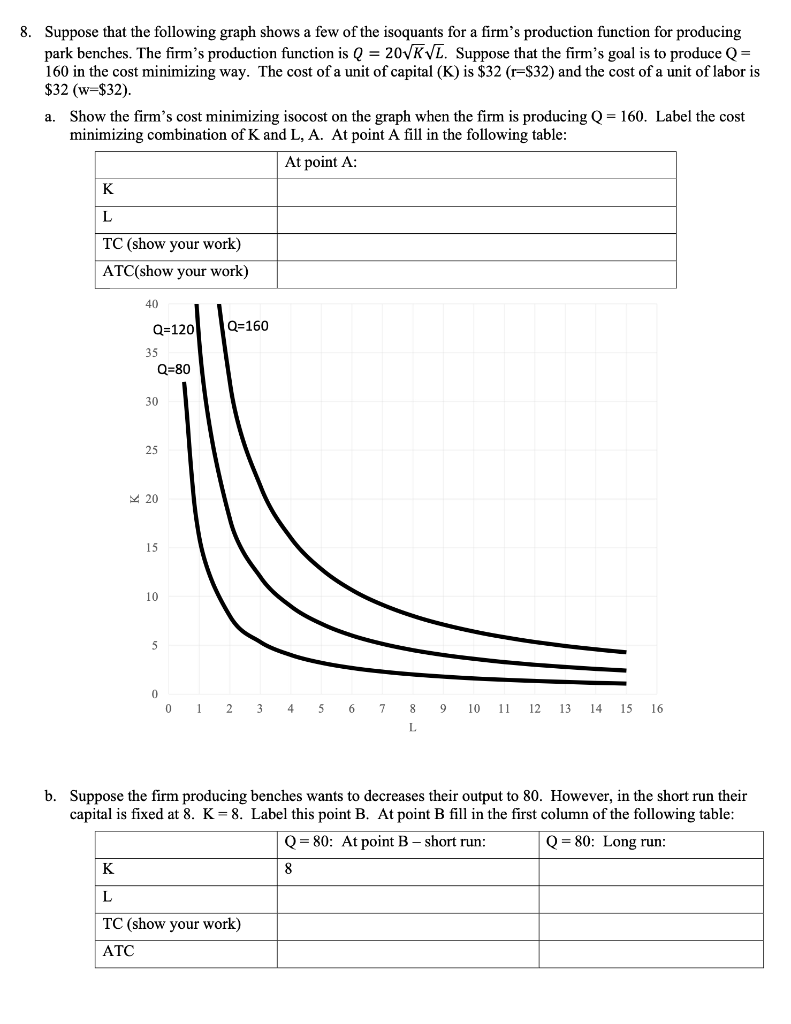8. Suppose that the following graph shows a few of the isoquants for a firm's production function for producing park benches. The firm's production function is Q = 20KVL. Suppose that the firm's goal is to produce Q= 160 in the cost minimizing way. The cost of a unit of capital (K) is \$32 (r=\$32) and the cost of a unit of labor is \$32 (w=\$32). a. Show the firm's cost minimizing isocost on the graph when the firm is...

Free Homework App